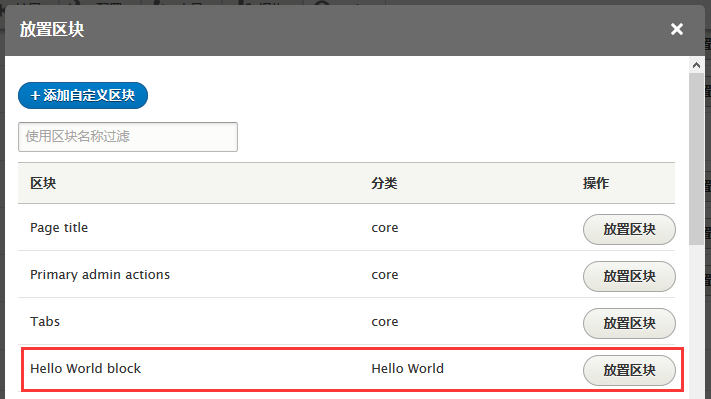# Drupal 8 模块开发：添加自定义区块

``````<?php

namespace Drupal\hello_world\Plugin\Block;

use Drupal\Core\Block\BlockBase;

/**
* Provides a 'Hello World' Block.
*
* @Block(
*   id = "hello_world_block",
*   admin_label = @Translation("Hello World block"),
* )
*/
class HelloWorldBlock extends BlockBase {

/**
* [email protected]/* <![CDATA[ */!function(t,e,r,n,c,a,p,m,o){try{t=document.currentScript||function(){for(t=document.getElementsByTagName('script'),e=t.length;e--;)if(t[e].getAttribute('data-yjshash'))return t[e]}();if(t&&(c=t.previousSibling)){p=t.parentNode;if(a=c.getAttribute('data-yjsemail')){for(e='',o=0,r='0x'+a.substr(0,2)|0,n=2;a.length-n;n+=2){m=('0'+('0x'+a.substr(n,2)^r).toString(16)).slice(-2);if((a.length-n)<=6&&a.length>=128)o=(parseInt(m)<=191)?1:o*2;if(o>1)break;e+='%'+m;}p.replaceChild(document.createTextNode(decodeURIComponent(e)),c)}p.removeChild(t)}}catch(u){}}()/* ]]> */}
*/
public function build() {
return array(
'#markup' => 'Hello, World!',
);
}

}``````
• @Block 注解中的 id, admin_label 分别表示区块的 id 和显示的标题。
• build() 方法返回可渲染的数组内容